## Symmetry and the Fourth Dimension (Part 9)

Last time in this series we completed the first phase of our quest: we got lots of polyhedra from Coxeter diagrams in a systematic way. But before we sail off into new seas, let’s review what we’ve done.

To spice things up, I’ll explain everything in a different way than before. If you don’t see how this new way relates to the old one, please ask! There’s a lot to say about this stuff that I’m not saying, so there are plenty of gaps left to fill.

And if you’re wondering what that thing is up there, read on.

### Coxeter theory

Harold Scott MacDonald Coxeter, the ‘king of geometry’, developed a beautiful theory relating groups to highly symmetrical polyhedra and their higher-dimensional generalizations, called ‘polytopes’.His book Regular Polytopes is required reading for anyone who wants to learn about these things. There’s a copy sitting by my bed, and there should be one by yours, too. It took him 24 years to write.

In his honor, finite groups generated by reflections in n-dimensional space are called Coxeter groups. They’re described by diagrams with n dots, called Coxeter diagrams. We’ve been looking at the 3d case, where there happen to be three such diagrams, with mysterious-sounding names:

A3      o—3—o—3—o
B3      o—3—o—4—o
H3      o—3—o—5—o

I want to show you to use these diagrams. So let’s do an example, namely A3:

o—3—o—3—o

This diagram is telling us to draw a bunch of triangles on a sphere, like this: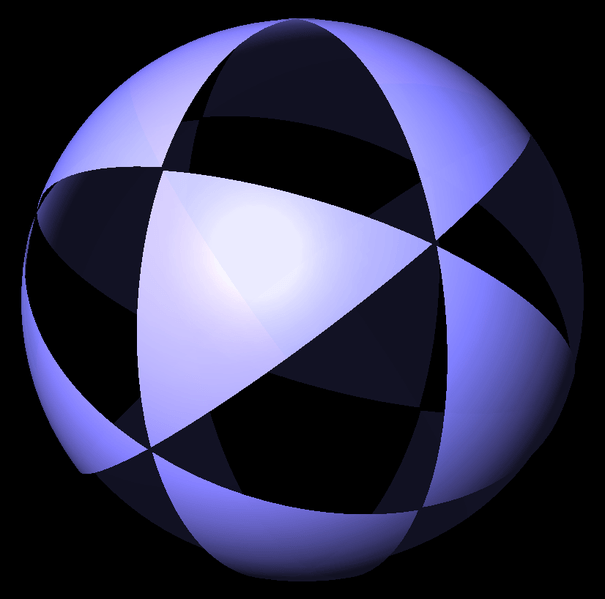This shape is called a Coxeter complex. As you can see, it contains lots of great circles. For each one we get a symmetry: the reflection across that circle, as if a mirror cut our Coxeter complex in half through that circle. So, the symmetries of the Coxeter complex form a finite group, generated by reflections. This is a Coxeter group!

But how do we get the Coxeter complex from the diagram? The dots in the diagram are secretly called V, E, and F:

V—3—E—3—F

Among other things, these letters are names for the edges of our favorite triangle in the Coxeter complex:It doesn’t matter which is our favorite triangle, so I just picked one. Each edge of this triangle gives a symmetry: the reflection that reflects the Coxeter complex across that edge! We call these symmetries V, E and F.

The 3 on the line going between V and E in this diagram:

V—3—E—3—F

is a quick way of saying that

(VE)3 = 1

In other words, doing the reflections V, E, V, E, V, E gets us back where we started.

To see this, let’s call our favorite triangle 1. Reflecting this triangle across the great circle containing the edge V, we get a new triangle, which we’ll call V. Reflecting that across the great circle containing the edge E, we get a new triangle, which we’ll call VE. And so on:By the time we get to VEVEVE = (VE)3, we’re back where we started! That’s a total of 6 reflections, so the V and E edges of each triangle must meet at a 60° angle.

Similarly, the 3 on the line going between E and V says that

(EF)3 = 1

so doing the reflections E, F, E, F, E, F also gets us back where we started:On the other hand, there’s no line connecting the dots V and F in our Coxeter diagram:

V—3—E—3—F

But this is an abbreviation for a line with a 2 on it! You see, lines with 2 on them occur so often that Coxeter decided to save time by not drawing such lines. So, we have

(VF)2 = 1

and doing the reflections V, F, V, F also gets us back where started: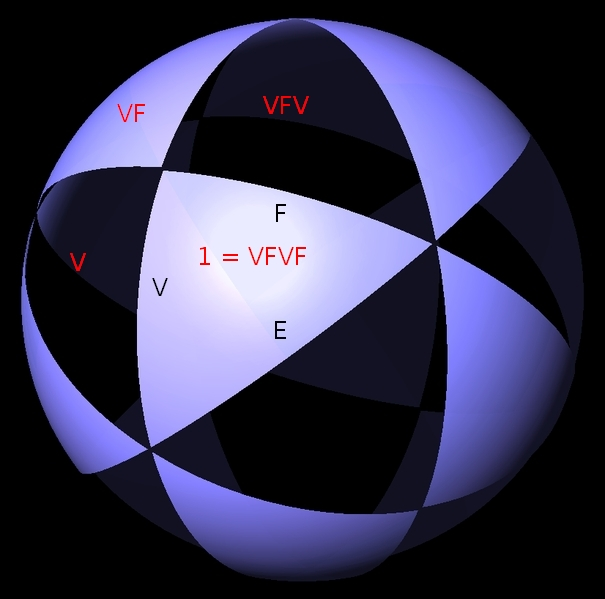That’s a total of 4 reflections, so the V and F edges of each triangle must meet at a 90° angle.

So, I’ve sketched how the Coxeter group and the Coxeter complex arise from the Coxeter diagram. To be a bit more precise, the Coxeter group A3 has generators V, E, F obeying relations

(VE)3 = (EF)3 = (VF)2 = 1

and also

V2 = E2 = F2 = 1

since V, E, and F are reflections. If we draw a sphere with one great circle serving as the ‘mirror’ for each reflection in the Coxeter group, we get the Coxeter complex.

What makes Coxeter complexes special, compared to other ways of tiling a sphere with triangles? One thing is that they have exactly as many symmetries as triangles. If you pick any triangle and call it your favorite, there’s exactly one symmetry—that is, rotation and/or reflection—sending this triangle to any other.

So, the Coxeter complex is actually a picture of its own symmetry group!

The particular Coxeter complex we’ve been looking at has 24 triangles. So, its symmetry group has 24 elements. This group is called the tetrahedral finite reflection group, or A3 for short. There’s a lot to say about it, but not now! There’s another more urgent question.

### Polyhedra

How do we get polyhedra from our Coxeter complex?

The easiest one works like this. We take the Coxeter complex and create a polyhedron with a corner in the middle of each triangle, connecting two corners with an edge whenever their triangles touch:The polyhedron looks better if we view it from a slightly different angle, and let a skilled artist like Tom Ruen do the drawing:This polyhedron is called the Poincaré dual of the Coxeter complex.

There’s a notation for this particular polyhedron:

•—3—•—3—•

All the dots are black, because this is the fanciest, most interesting polyhedron that comes from our Coxeter complex. We get other polyhedra by blackening just some of the dots.

Earlier in this series, I described how to build these other polyhedra using the concept of ‘flag’. But there’s another way, using the Coxeter complex, which I’ll sketch here.

Suppose we blacken just some of the dots in our Coxeter diagram. Then our favorite triangle belongs to a bunch of triangles, all related by reflections corresponding to the dots we left white. And indeed, all the triangles can be grouped into bunches that all look the same… and there’s a polyhedron that has a corner in the middle of each bunch of triangles.

For example, suppose we leave the E dot white:

•—3—o—3—•

Then our favorite triangle belongs to a bunch of triangles—in this case, just a pair!—that are related by reflections along the E edge of our favorite triangle. If we group our triangles into pairs like this, we get a polyhedron with a corner in the middle of each pair:Again, it looks better if we let Tom Ruen draw it: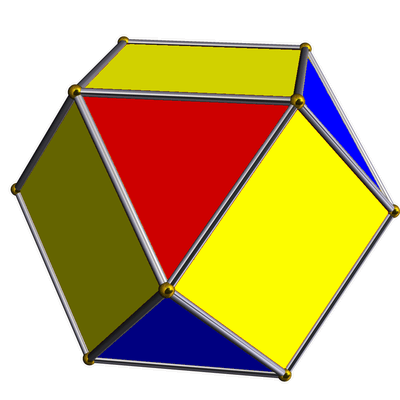I should do more examples, but I think I’ll wrap up by describing the procedure in more highbrow language. Skip the next paragraph if you don’t know group theory, and move on to the complete list of examples!

If we call the Coxeter group G, blackening all the dots of the Coxeter diagram gives a polyhedron with one corner for each element of G. But if we don’t blacken all of them, the reflections corresponding to the white dots generate a subgroup B of G. Then we get a polyhedron with one corner for each element of G/B. Last time I said each way of blackening some dots describes some sort of ‘flag’. In these terms, B is the subgroup fixing your favorite flag of this sort, and G/B is the set of all flags of this sort. Each of those flags corresponds to what I’m calling a ‘bunch of triangles’ in my current story.

But now let’s see what we get from all this! We get three families of polyhedra, and these are almost all the ‘Archimedean solids’ in 3 dimensions.

### The A3 family:     o—3—o—3—o

This family of polyhedra can all be gotten from the tetrahedron by chopping off vertices, edges or faces. They’re associated to the Coxeter complex we’ve just been looking at:It’s built from triangles whose interior angles are$\pi/3, \pi/3$ and$\pi/2$. These numbers come from taking π and dividing it by the numbers on the edges of the Coxeter diagram: 3, 3, and the invisible edge labelled 2.

As mentioned, this Coxeter complex has 24 triangles, and its symmetry group, with 24 elements, is called the tetrahedral finite reflection group, or A3.

Here are all the polyhedra in this family. The list has some repeats, because this Coxeter diagram is its own mirror image!

 tetrahedron•—3—o—3—o truncated tetrahedron•—3—•—3—o octahedron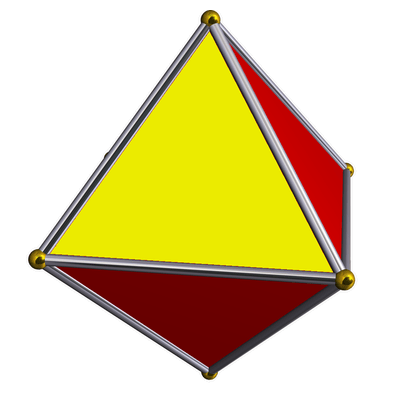o—3—•—3—o truncated tetrahedrono—3—•—3—• tetrahedron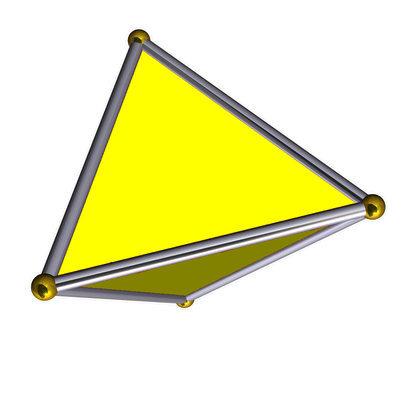o—3—o—3—• cuboctahedron•—3—o—3—• truncated octahedron•—3—•—3—•

### The B3 family:     o—3—o—4—o

This family of polyhedra can all be gotten by taking the cube or the octahedron and chopping off vertices, edges or faces. They come from the Coxeter complex whose triangles have interior angles$\pi/3, \pi/4$ and$\pi/2$: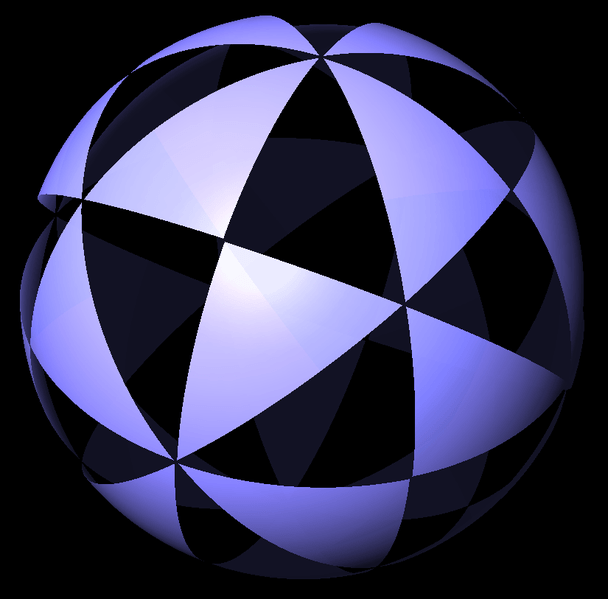This Coxeter complex has 48 triangles, and its symmetry group is a Coxeter group with 48 elements, called the octahedral finite reflection group, or B3.

Here are all the polyhedra in this family. We’ve seen some of these already in the A3 family, and there’s a reason for that: the group B3 happens to contain A3 as a subgroup! It’s twice as big.

 cube•—4—o—3—o truncated cube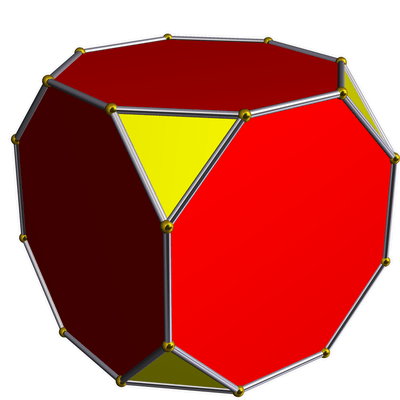•—4—•—3—o cuboctahedrono—4—•—3—o truncated octahedrono—4—•—3—• octahedrono—4—o—3—• rhombicuboctahedron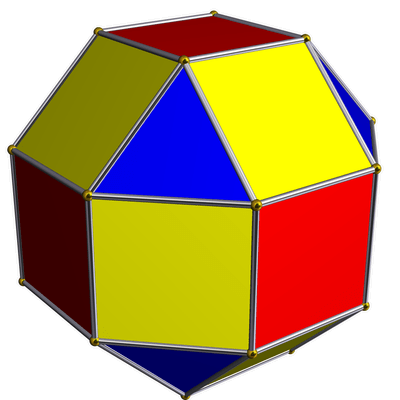•—4—o—3—• truncated cuboctahedron•—4—•—3—•

### The H3 family:     o—3—o—5—o

This family of polyhedra can all be gotten by taking the dodecahedron or icosahedron and chopping off vertices, edges and faces. They come from the Coxeter complex built from triangles whose interior angles are$\pi/3, \pi/5$ and$\pi/2$:This Coxeter complex has 120 triangles, and its symmetry group is a Coxeter group with 120 elements, called the icosahedral finite reflection group, or H3.

Here are all the polyhedra coming from this Coxeter complex. These are my favorites, because they look the most fancy, and I have a fondness for the quirky charm of 5-fold symmetry:

 dodecahedron•—5—o—3—o truncated dodecahedron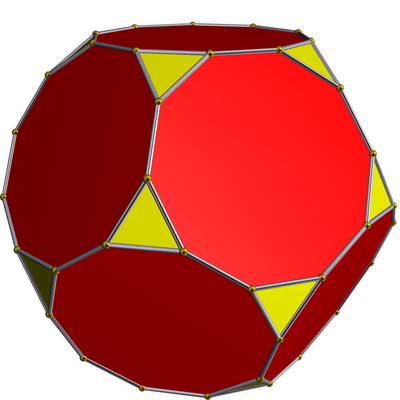•—5—•—3—o icosidodecahedrono—5—•—3—o truncated icosahedron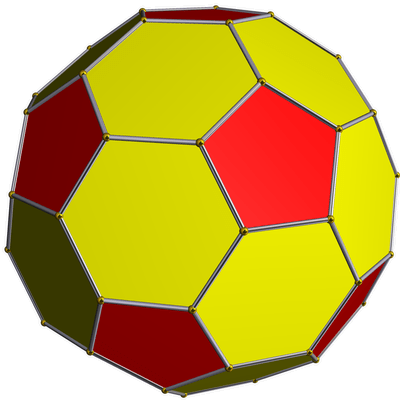o—5—•—3—• icosahedrono—5—o—3—• rhombicosidodecahedron•—5—o—3—• truncated icosidodecahedron•—5—•—3—•

### Afterword

The picture at the start of this post was taken by my friend Allen Knutson:

It’s the H3 Coxeter complex, and for some reason it’s in the music library at Cornell University, where Allen teaches math.

The picture of H. S. M. Coxeter is from the cover of a book about him:

• Siobhan Roberts, King of Infinite Space, Walker & Company, 2006.

As usual, the pretty pictures of solids with brass balls at the vertices were made by Tom Ruen using Robert Webb’s Stella software. Tom Ruen also drew the Coxeter complexes; I’m to blame for the crude extra stuff added on.

You can see the previous episodes of this series here:

Part 1: Platonic solids and Coxeter complexes.

Part 2: Coxeter groups.

Part 3: Coxeter diagrams.

Part 4: duals of Platonic solids.

Part 5: Interpolating between a Platonic solid and its dual, and how to describe this using Coxeter diagrams. Example: the cube/octahedron family.

Part 6: Interpolating between a Platonic solid and its dual. Example: the dodecahedron/icosahedron family.

Part 7: Interpolating between a Platonic solid and its dual. Example: the tetrahedron family.

Part 8: The missing solids, coming from Coxeter diagrams with both ends blackened.

### 10 Responses to Symmetry and the Fourth Dimension (Part 9)

1. We’ve been talking about nice 4-dimensional shapes: the 4d relatives of the Platonic and Archimedean solids. In the series so far, we’ve spent a lot of time warming up by studying the 3d Platonic and Archimedean solids […]

2.Phil Gossett says:

An interesting pattern:

If you start with the symmetrical Coxeter diagrams for the A3 family, and “shift right”, you get the Coxeter diagrams for the B3 family with a “leading zero” (white dot in the V position) representing the same polyhedron:

•—3—o—3—•   →   o—4—•—3—o   cuboctahedron

•—3—•—3—•   →   o—4—•—3—• truncated octahedron

o—3—•—3—o   →   o—4—o—3—• octahedron

The other (asymmetrical) A3 diagrams (for tetrahedron and truncated tetrahedron) pair within the A3 family.

So all members of the B3 family with a white dot in the V position pair with a member of the A3 family. And all members of the A3 family pair with something. And these seem to cover all the duplications of polyhedra in these families.

I wonder if something like this pattern extends to higher dimensions…

•John Baez says:

Thanks for prodding me to think harder about the relation between the A3 family and B3 family. It will take me a while to fully work it out, but it will be fun.

Mathematically, the relation starts because the symmetry group of the cube contains the symmetry group of the tetrahedron. The symmetry group of the tetrahedron has 4! = 24 elements since you can permute its vertices any way you like. The symmetry group of the cube has 23 × 3! = 48 elements since you can reflect it along any 3 of the coordinate axes and also permute these axes any way you like. 48 is a multiple of 24, so there’s an opportunity for the symmetry group of the cube to contain the symmetry group of the tetrahedron. Nature exploits this opportunity. You can see it here:

where we fit two tetrahedra in a cube, getting a shape Kepler called the stella octangula:

This magic doesn’t happen in higher dimensions! In n dimensions the symmetry group of the n-simplex (the analogue of a tetrahedron) has (n+1)! elements. The symmetry group of the n-cube has n! × 2n elements. And I think the former is never a subgroup of the latter. I guess we can see this by a simple divisibility argument.

For example, in 4 dimensions we get 120 elements in the first group, 24 × 16 = 384 in the second. Since 384 isn’t a multiple of 120, the symmetry group of the 4-cube doesn’t contain the symmetry group of the 4-simplex.

The first group is called the An Coxeter group; the second is called the Bn Coxeter group.

So, I think we’re lucky in 3 dimensions.

•Phil Gossett says:

Thank you for pointing out the counting argument. Makes perfect sense why it’s possible in 3D but not in 4D.

I don’t want to distract folks from the path you’re on with 4D rotations and such. But I think I figured out why this works (in 3D). And found a similar pattern in 4D (though not the one I was expecting).

It seems that this works because an octahedron happens to be the same thing as a rectified tetrahedron. Once you have established that a parent X = a rectified Y, then a truncated X = a cantitruncated Y, and a rectified X = a cantellated Y. Which is (in words) the above pattern.

This is more-or-less obvious from:

http://en.wikipedia.org/wiki/Uniform_polytope

And in particular if you stare long enough at:The (unexpected) pattern in 4D is between the B4 and F4 families (the latter being the 24 cell family, unique to 4D):

o—•—o==o   →   •—o==o—o
rectified 16 cell → 24 cell

•—•—•==o   →   •—•==o—o
cantitruncated 16 cell → truncated 24 cell

•—o—•==o   →   o—•==o—o
cantellated 16 cell → rectified 24 cell

(In the interests of sloth, I’m abusing notation somewhat, borrowing from undirected Dynkin diagrams: a double line to denote -4-, and a single line to denote -3-.)

Just as with an octahedron and a rectified tetrahedron, this depends on a 24 cell being the same thing as a rectified 16 cell. Which I guess is just a coincidence.

Though it’s interesting that it carries over so directly. A 16 cell is, after all, a 4D octahedron. And if you just drop the final —o from the above, and you get that same 3D pattern.

•John Baez says:

Wow, this is great! My ultimate goal is to go through all the polytopes coming from 4-dot Dynkin diagrams, and say a lot of fun things about them. I hadn’t noticed these relations between the B4 and F4 series! But now I plan to talk about them when I reach this point.

The 24-cell is one of the wonders of nature, so it’s especially nice to see it partaking in these relations.

It’s also great to see how the 3d relations you noticed earlier are a kind of spinoff of these 4d ones.

Fun, fun, fun…

3.Tom Ruen says:

Good stuff! :) Don’t forget all this extends out into the regular and uniform Euclidean and Hyperbolic plane tilings too!
http://en.wikipedia.org/wiki/Uniform_tiling
http://en.wikipedia.org/wiki/Uniform_tilings_in_hyperbolic_plane
Plus 3D “Honeycombs”, filling space by polyhedra, created from 4-mirror kaleidoscopes:
http://en.wikipedia.org/wiki/Convex_uniform_honeycomb
http://en.wikipedia.org/wiki/Uniform_honeycombs_in_hyperbolic_space
http://en.wikipedia.org/wiki/Paracompact_uniform_honeycombs
And on the hyperbolic front, Italian wikipedian Claudio Rocchini has helped with lots of graphics, including the hyperbolic regular hyperbolic honeycomb {6,3,3}, 3 hexagonal tilings wrapped around an edge, never rendered before as best I know!
http://en.wikipedia.org/wiki/User:Rocchini4.Jeffrey Haemer says:

In the VE-labelled Coxeter complex, are the “VEVE” and “VE” triangle labels swapped? (Ditto “EF” and “EFEF” in the following one.)

•John Baez says:

I think I labelled them correctly according to the conventions I’m describing in this post. For example, here:the triangle labelled “VE” is obtained from the triangle labelled “V” by reflecting it across the great circle containing the edge labelled “E”. Then the triangle labelled “VEV” is obtained from the triangle labelled “VE” by reflecting it across the great circle containing the edge labelled “V”. And so on.

You may be confused because these conventions are different than the conventions I used in Part 2. There, instead of repeatedly reflecting a triangle across fixed great circles, I kept flipping it over one of its edges, each time getting a new triangle that shared one edge with the previous triangle: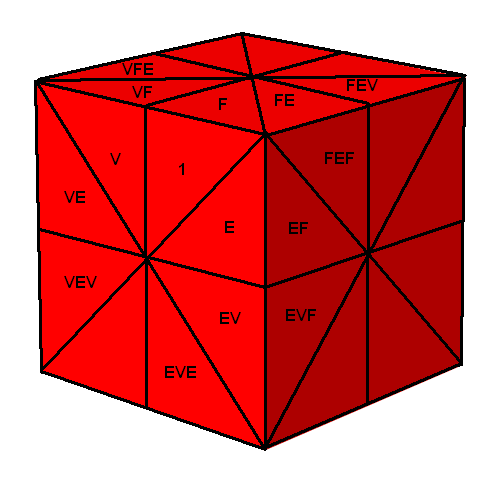Both these approaches are useful; they’re different, but related in an interesting way. I remember Greg Egan getting confused when I used the first approach when he was expecting the second one… and now I’m thinking maybe you’re confused because I’m using the second approach when you were expecting the first one. Is that right?

Clearly I should have done a better job warning everyone that I was talking about things a new way this time! And someday I should explain how the two ways are related (though Greg figured it out).

•Jeffrey Haemer says:

Yup. That’s what I was thinking. You don’t need to warn better, you just need to get readers who pay closer attention. :-)

5. Marking the Coxeter diagram in different ways lets us describe many honeycombs with the same symmetry group as the hexagonal tiling honeycomb—in fact,$2^4 - 1 = 15$ of them, since there are 4 dots in the Coxeter diagram! For the theory of how this works, illustrated by some simpler examples, try this old post of mine…

This site uses Akismet to reduce spam. Learn how your comment data is processed.